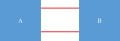# Does an impedance matching concern equivalent impedances of circuits or individual impedances?

#### Dong-gyu Jang

Joined Jun 26, 2015
115
Hello.

Let's see my attached image first.There are two circuits A and B which are connected by red lines (red lines forms a port). Obviously there are two electronic components in A and B which are diirectly connected by the red lines; they are called Ca and Cb respectively and are the very ends of the red lines. Let's call individual impedances of these components Za and Zb respectively and ZaZb. However, equivalent impedance of circuits A and B are matched.

Then, is there a signal reflection when the signal transfers from A to B? What does the signal actually see? Does it concern impedances of very end individual components of the line on which it travels or equivalent impedances of whole circuits at both side of the line?

I need to know this in order to take a step toward a understanding of the impedance matching network